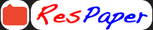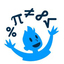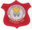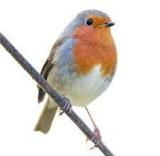Trending ▼   ResFinder# ICSE Class IX Prelims 2022 : Mathematics (Saint Lawrence School (SLS), Tentoloi, Balaramprasad, Angul)

3 pages, 34 questions, 2 questions with responses, 2 total responses,20Anil Kumar Dash Saint Lawrence School (SLS), Tentoloi, Balaramprasad, Angul
+Fave Message
 Home > 9938480846 >   F Also featured on: School Page icse9Formatting page ...

Class 9 Saint Lawrence School, Tentoloi Half Yearly Examination 2021 Sub- Mathematics Date- 05.10.2021 Time 8am to 10am F.M - 80 -:General Instructions:1. Attempt all questions from section A and any four questions from section B. 2. All working including rough work must be clearly shown and must be done on the same sheet as the rest of the answer. 3. Omission of essential working will result in the loss of marks. 4. The intended marks for questions as parts of questions are given in brackets [ ] Section-A (40 marks) Q1). Q2). Q3). Q4). (a) Solve for x & y by substitution method. 3x - 7= & x + = 1 (b). The area of circular ring enclosed between two concentric is 286 cm2. Find the radii of the two circles given that their difference is 7 cm. (c). Factories the following. (i) (x2 - x) (4x2 - 4x - 5) - 6 (ii) a3 - a - 120 1 (a) If x=2+ , find the value of + x (b). Calculate the interest Rs 6000 in 3 years at 5% per annum compounded annually. (Without using formula) (c). In an isosceles ABC with AB=AC, the bisectors of B & C intersect each other at O. Show that : (i) OB = OC (ii) AO bisects A 1 1 1 2 2 (a) If ab + bc + ca = 0, Then find the value of :2 a bc b ac c ab (b). The ratio of incomes of two persons is 9:7 & the ratio of their expenditures is 4:3. If each of them manages to save Rs 2000 per month. Find their monthly incomes. (c). If O is any point in the interior of ABC prove that :- OA+OB+OC < AB+BC+CA 1 3 1 (a) If x2 + = 8 , Find x3 + 2 5 25 x 125 x 3 (b). The base of a triangular field is 3 times its height. If the cost of cultivating the field at the rate of Rs25 per 100m2 is Rs60,000. Find its base& the height. (c). ABCD is a parallogram.. E & F are the mid points of the sides AB & CD. Prove that AF & CE trisect the diagonal BD.Formatting page ...

Top Contributors
to this ResPaperResPaper Admins(1)Bob Bird(1)Shashwat Shaw 9H 35(1)Formatting page ...